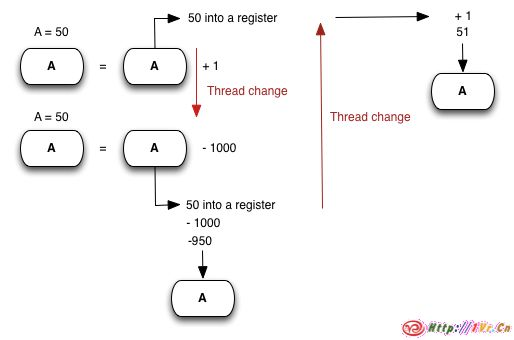# 了解Unity中的多线程及使用多线程

Unity编程时,总有个主线程执行你的代码,也可以创建额外的线程和主线程同时运行.C#中有lock这个关键字,以确保只有一个线程可以在特定时间内访问特定的对象.这里说对象是因为无法锁定一个类型值(value type)或原型(primitive).

`int a = 50;`

``` object guard = new object(); void ThreadOneCode() { //一些代码在这 lock(guard) { a = a + 1; } //其余一些代码在这 } void ThreadTwoCode() { //一些代码在这 lock(guard) { a = a - 1000; } ```

```//其余一些代码在这 } ```

RunAsync(Action)-在另一个线程上运行的一组代码.

```//缩放一个网格在第二个线程 void ScaleMesh(Mesh mesh, float scale) { //Get the vertices of a mesh var vertices = mesh.vertices; //运行一个Action在新的线程 Loom.RunAsync(()=>{ //遍历所有的顶点 for(var i = 0; i < vertices.Length; i++) { //缩放顶点 vertices[i] = vertices[i] * scale; } //在主线程上运行一些代码 //更新网格 Loom.QueueOnMainThread(()=>{ //设置顶点 mesh.vertices = vertices; //重新计算边界 mesh.RecalculateBounds(); }); ```

```//缩放一个网格在第二个线程 function ScaleMesh(mesh : Mesh, scale : float) { //Get the vertices of a mesh var vertices = mesh.vertices; //运行一个Action在新的线程 Loom.RunAsync(function() { //遍历所有的顶点 for(var i = 0; i < vertices.Length; i++) { //缩放顶点 vertices[i] = vertices[i] * scale; } //在主线程上运行一些代码 //更新网格 Loom.QueueOnMainThread(function() { //设置顶点 mesh.vertices = vertices; //重新计算边界 mesh.RecalculateBounds(); }); ```